# Finding Maximum of Two Numbers in C++

## Finding Maximum of Two Numbers Program in C++:

In this article, I am going to discuss How to Find the Maximum of Two Numbers Program in C++ with Examples. Please read our previous article where we discussed Conditional Statements in C++ with Examples.

##### Finding Maximum of Two Numbers:

Here, we will write a program using conditional statements which will find the largest of two numbers. Suppose we have two numbers ‘x’ and ‘y’, then we have to find out which one is greater. So, it’s very simple. We have to compare them. So, we have to just check the condition that is if (x > y). So, for writing this program, we will follow the same steps that we have used.These are steps that are taking input, processing, and giving output. So, in this program, ‘x’ and ‘y’ are the input. Then we have to check the condition i.e. if (x > y) which is processing and then give the output which will be either ‘x’ or ‘y’. Let us draw a flow chart for Finding the Maximum of Two Numbers and then we will write the algorithm followed by the program.

##### Flowchart for checking the Largest Number:

1st step, we start the flowchart.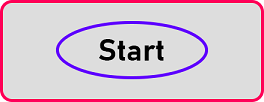2nd step, we take two numbers as input.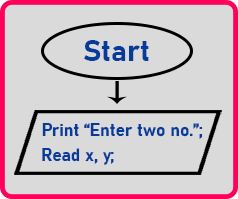Here we used the input/ output box and print ‘Enter two no.’ Then we read the numbers. So here I have used ‘x’ and ‘y’ variables. After reading these values we have to check which one is greater.

3rd step.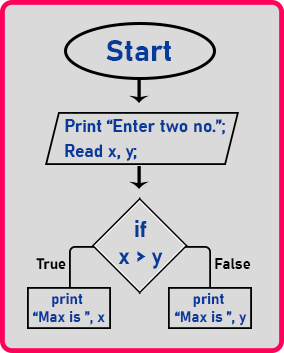Now inside if condition, if x is greater than y then it will true and take left direction and print “Max is x” otherwise if it is false then it will take the right direction and print “Max is y”. Here simply we will display the largest number. Then after this, the output is over. So, in this example, there is no processing right. Then after this, we will end the flowchart.

4th step.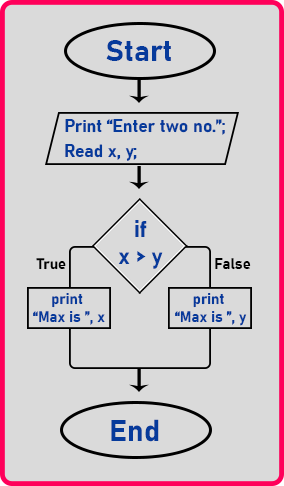This is the flow chart for finding the greatest or maximum of two numbers. Now the same thing we will write it as an algorithm.

##### Algorithm for Finding Maximum of Two Numbers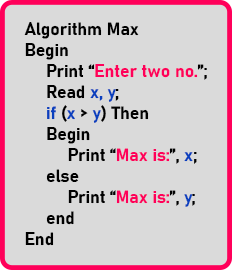This is the pseudocode for finding the maximum of two numbers. Now next we will write a C++ program for this.

##### Finding Maximum of 2 Numbers Program Code in C++:
```#include <iostream>
using namespace std;
int main()
{
int x, y;

cout << "Enter two numbers: ";
cin >> x >> y;

if (x > y)
{
cout << "Max is " << x;
}
else
{
cout << "Max is " << y;
}

return 0;
}
```
###### Output: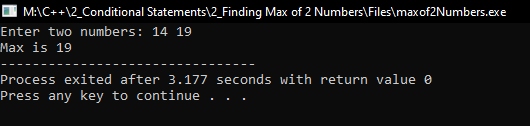In the next article, I am going to discuss Logical Operators in C++ with Examples. Here, in this article, I try to explain How to Find Maximum of Two Numbers Program in C++ with Examples and I hope you enjoy this Finding Maximum of Two Numbers Program in C++ with Examples article.